Over at the SAS and R blog, Ken Kleinman discussed using polar coordinates to plot time series data for multiple years. The time series plot was reproduced in SAS by my colleague Robert Allison.

The idea of plotting periodic data on a circle is not new. In fact it goes back at least as far as Florence Nightingale who used polar charts to plot the seasonal occurrence of deaths of soldiers during the Crimean War. Her diagrams are today called "Nightingale Roses" or "Coxcombs."

The polar charts created by Kleinman and Allison enable you to see general characteristics of the data and could be useful for comparing the seasonal temperatures of different cities. A city such as Honolulu, Hawaii, that has a small variation in winter-summer temperatures will have a polar diagram for which the data cloud is nearly concentric. Cities with more extreme variation—such as the Albany, NY, data used by Kleinman and Allison—will have a data cloud that is off center. Comparing cities by using polar charts has many of the same advantages and disadvantages as using radar charts.

However, if you want to model the data and display a fitted curve that shows seasonality, then a rectangular coordinate system is better for displaying the data. For me, trying to follow a sinusoidal curve as it winds around a circle requires too much head-tilting!

### Fitting periodic data: The quick-and-dirty way

You can visualize periodic time-series data by "folding" the data onto a scatter plot. The easiest way to do this is to plot the day of the year for each data point. (The day of the year is called the "Julian day" and is easily computed by applying the SAS JULDAYw format.) That produces a scatter plot for which the horizontal axis is in the interval [1, 365], or [1, 366] for leap years. An alternative approach is to transform each date into the interval (0,1] by dividing the Julian day by the number of days in the year (either 365 or 366). The following SAS code performs a "quick and dirty" fit to the temperature data for Albany, NY. The code does the following:

• A DATA step reads the temperatures for Albany, NY, from its Internet URL. This uses the FILENAME statement to access data directly from a URL.
• The Julian day is computed by using the JULDAY3. format.
• The proportion of the year is computed by dividing the Julian day by 365 or 366.
• The winter of 2011-2012 is appended to the data to make it easier to accent those dates while using transparency for the bulk of the data.
• The SGPLOT procedure is used to create a scatter plot of the data and to overlay a loess curve.

```/* Read the data directly from the Internet. This DATA step adapted from Robert Allison's analysis: http://sww.sas.com/~realliso/democd55/albany_ny_circular.sas */ filename webfile url "http://academic.udayton.edu/kissock/http/Weather/gsod95-current/NYALBANY.txt" /* behind a corporate firewall? don't forget the PROXY= option here */ ; data TempData; infile webfile; input month day year Temperature; format date date9.; date=MDY(month, day, year); dayofyear=0; dayofyear=put(date,julday3.); /* incorporate leap years into calculations */ Proportion = dayofyear / put(mdy(12, 31, year), julday3.); label Proportion = "Day of Year (Normalized)"; CurrentWinter = (date >='01dec2011'd & date<='15mar2012'd); if Temperature^=-99 then output; run;   /* Technique to overlay solid markers on transparent markers */ data TempData; set TempData /* full data (transparent markers) */ TempData(where=(CurrentWinter=1) /* special data (solid) */ rename=(Proportion=Prop Temperature=Temp)); run;   proc sgplot data=TempData; scatter x=Proportion y=Temperature / transparency=0.8; scatter x=Prop y=Temp / markerattrs=(color='gray' symbol=CircleFilled) legendlabel="Winter 2011-12"; loess x=Proportion y=Temperature / smooth=0.167 nomarkers lineattrs=(thickness=4px) legendlabel="Loess smoother"; yaxis grid; title "Temperature in Albany, NY (1995-2012)"; run;```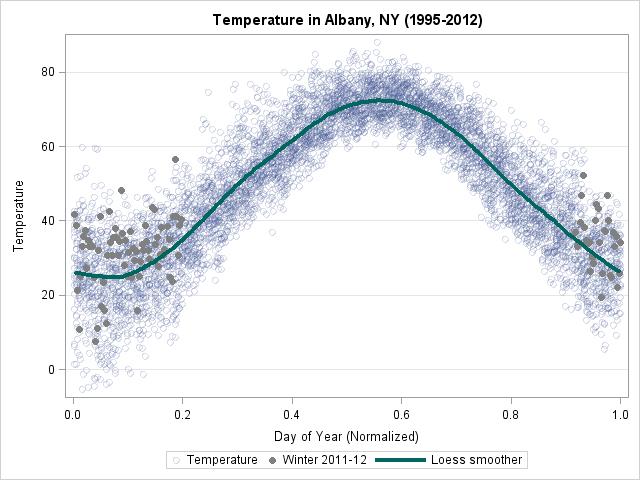A few aspects of the plot are interesting:

• Only about 27% of this past winter's temperatures are below the average temperature, as determined by the loess smoother. This indicates that the Albany winter was warmer than usual—a result that was not apparent in the polar graph.
• The smoother enables you to read the average temperatures for each season.

The observant reader might wonder about the value of the smoothing parameter in the LOESS statement. The smoothing value is 61/365 = 0.167, which was chosen so that the mean temperature of a date in the center of the plot is predicted by using a weighted fit of temperatures for 30 days prior to the date and 30 days after the date. If you ask the LOESS procedure to compute the smoothing value for these data according to the AICC or GCV criterion, both criteria tend to oversmooth these data.

### Creating a periodic smoother

The loess curve for these data is very close to being periodic....but it isn't quite. The value at Proportion=0 and Proportion=1 are almost the same, but the slopes are not. For other periodic data that I've examined, the fitted values have not been equal.

Why does this happen? Because of what are sometimes called "edge effects." The loess algorithm is different near the extremes of the data than it is in the middle. In the middle of that data, about k/2 points on either side of x are used to predict the value at x. However, for observations near Proportion=0, the algorithm uses about k points to the right of x, and for observations near Proportion=1, the loess algorithm uses about k points to the left of x. This asymmetry leads to the loess curve being aperiodic, even when the data are periodic.

Tomorrow I will show how to create a really, truly, honestly periodic smoother for these data.Over at the SAS Discussion Forums, someone asked how to use SAS to fit a Poisson distribution to data. The questioner asked how to fit the distribution but also how to overlay the fitted density on the data and to create a quantile-quantile (Q-Q) plot.

The questioner mentioned that the UNIVARIATE procedure does not fit the Poisson distribution. That is correct: the UNIVARIATE procedure fits continuous distributions, whereas the Poisson distribution is a discrete distribution. Nevertheless, you can fit Poisson data and visualize the results by combining several SAS procedures. This article shows one way to accomplish this. The method also works for other discrete distributions such as the negative binomial and the geometric distribution.

### Do I receive emails at a constant rate?

For data I will use the number of emails that I received one day for each of 19 half-hour periods from 8:00 am to 5:30 pm. If I receive emails at a constant rate during the day, the number of emails in each 30-minute period follows a Poisson distribution. The following DATA step defines the data; PROC FREQ tabulates and plots the sample distribution:

```/* number of emails received in each half-hour period 8:00am - 5:30pm on a weekday. */ data MyData; input N @@; /* 8a 9a 10a 11a 12p 1p 2p 3p 4p 5p */ datalines; 7 7 13 9 8 8 9 9 5 6 6 9 5 10 4 5 3 8 4 ; run; /* Tabulate counts and plot data */ proc freq data=MyData; tables N / out=FreqOut plots=FreqPlot(scale=percent); run;```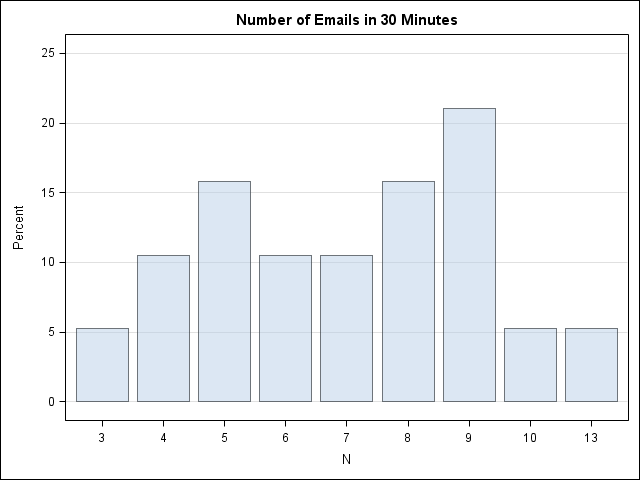The mean of the data is about 7. A Poisson(7) distribution looks approximately normal—which these data do not. On the other hand, there are less than 20 observations in the data, so let's proceed with the fit. (I actually looked at several days of email before I found a day that I could model as Poisson, so these data are NOT a random sample!)

### Fit the data

The first step is to fit the Poisson parameter to the data. You can do this in PROC GENMOD by by using the DIST= option to specify a Poisson distribution. Notice that I do not specify any explanatory variables, which means that I am fitting the mean of the data.

```/* 1. Estimate the rate parameter with PROC GENMOD: http://support.sas.com/kb/24/166.html */ proc genmod data=MyData; model N = / dist=poisson; output out=PoissonFit p=lambda; run;```

At this point you should look at the goodness-of-fit and parameter estimates tables that PROC GENMOD creates to see how well the model fits the data. I will skip these steps.

### Compute the fitted density

The P= option on the OUTPUT statement outputs the mean, which is also the parameter estimate for the fitted Poisson distribution. The mean is about 7.1. The following statements set a macro variable to that value and create a data set (PMF) that contains the Poisson(7.1) density for various x values. In a subsequent step, I'll overlay this fitted density on the empirical density.

```/* 2. Compute Poisson density for estimated parameter value */ /* 2.1 Create macro variable with parameter estimate */ data _null_; set PoissonFit; call symputx("Lambda", Lambda); stop; run;   /* 2.2 Use PDF function for range of x values */ data PMF; do t = 0 to 13; /* 0 to max(x) */ Y = pdf("Poisson", t, &Lambda); output; end; run;```

### Overlay the empirical and fitted densities

I want to overlay the discrete density on a bar chart of the data. One way to visualize the discrete density is as a scatter plot of (x, pdf(x)) values that represent the fitted density at x=0, 1,...,13. Unfortunately, you cannot use the VBAR and the SCATTER statements in the same SGPLOT call to overlay a bar chart and a scatter plot. However, in SAS 9.3 you can use the VBARPARM statement together with the SCATTER statement. (Thanks to "PGStats" for this suggestion.) The VBARPARM statement requires that you compute the heights of the bars yourself, but the heights are easily constructed from the PROC FREQ output that was created earlier:

```/* 3. Use bar chart to plot data. To overlay a bar chart and scatter plot, use the VBARPARM stmt instead of VBAR. */ data Discrete; merge FreqOut PMF; Prop = Percent / 100; /* convert to same scale as PDF */ run;   /* 3.2 Overlay VBARPARM and scatter plot of (x, pdf(x)) */ proc sgplot data=Discrete; /* VBARPARM is SAS 9.3 stmt */ vbarparm category=N response=Prop / legendlabel='Sample'; scatter x=T y=Y / legendlabel='PMF' markerattrs=GraphDataDefault(symbol=CIRCLEFILLED size=10); title "Emails per 30-Minute Period and Poisson Distribution"; run;```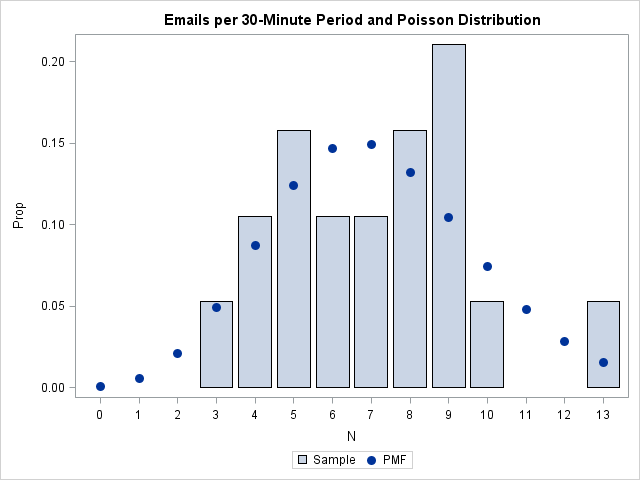### Create a discrete Q-Q plot

On the Discussion Forum, the questioner asked for a quantile-quantile plot. I don't know whether I've ever seen a Q-Q plot for a discrete distribution before; usually they are shown for continuous distributions. However, you can create a discrete Q-Q plot by following exactly the same steps that I described in my previous article on how to compute a Q-Q plot:

```/* 4. Create a Q-Q plot */ /* 4.1 Compute theoretical quantiles */ proc sort data=MyData; by N; run; /* 1 */ data QQ; set MyData nobs=nobs; v = (_N_ - 0.375) / (nobs + 0.25); /* 2 */ q = quantile("Poisson", v, &Lambda); /* 3 */ run;   proc sgplot data=QQ noautolegend; /* 4 */ scatter x=q y=N; lineparm x=0 y=0 slope=1; /* SAS 9.3 statement */ xaxis label="Poisson Quantiles" grid; yaxis label="Observed Data" grid; title "Poisson Q-Q Plot of Emails"; run;```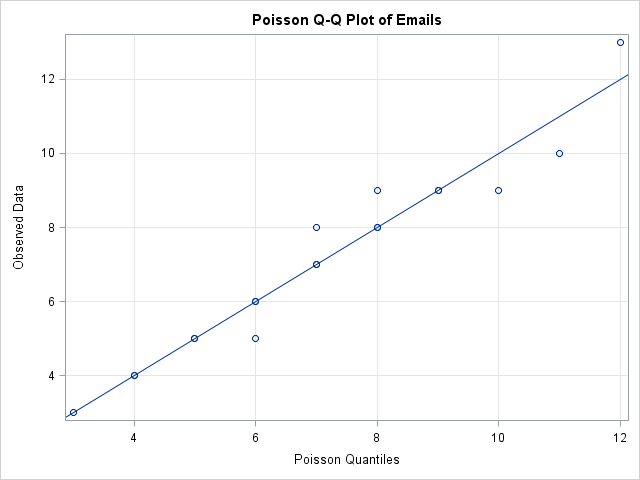I've created a discrete Q-Q plot, but is it useful? A drawback appears to be that the discrete Q-Q plot suffers from overplotting, whereas a continuous Q-Q plot does not. A continuous CDF function is one-to-one so the quantiles of the ranks of the data are unique. In contrast, the CDF function for a discrete distribution is a step function, which leads to duplicated quantiles and overplotting.

For example, in the discrete Poisson Q-Q plot for my email, there are 19 observations, but only 13 points are visible in the Q-Q plot due to overplotting. If I analyze 10 days of my email traffic, I could get 190 observations, but the Q-Q plot might show only a fraction of those points. (In simulated data, there were only 25 unique values in 190 observations drawn from a Poisson(7) distribution.)

The fact that I don't often see discrete Q-Q plots bothered me, so I did a little research. I found a reference to discrete Q-Q plots on p. 126 of Computational Statistics Handbook with MATLAB where it says:

Quantile plots...are primarily used for continuous data. We would like to have a similar technique for graphically comparing the shapes of discrete distributions. Hoaglin and Tukey  developed several plots to accomplish this [including] the Poissonness plot.

That sounds interesting! A future blog post will present an alternative way to visualize the fit of a Poisson model.Locating missing values is important in statistical data analysis. I've previously written about how to count the number of missing values for each variable in a data set. In Base SAS, I showed how to use the MEANS or FREQ procedures to count missing values. In the SAS/IML language, I demonstrated the COUNTN and COUNTMISS functions that were introduced in SAS/IML 9.22.

But did you know that you can also use the COUNTN and COUNTMISS functions to determine the number of missing values in each observation? This can be useful in an analysis (such as regression) in which you need to delete observations that contain one or more missing value. Or it can be useful to determine observations that contain missing values for a large number of variables.

To begin, let's count missing values in the SAS DATA step by using the CMISS function.

### Count missing values in the DATA step

For the SAS DATA step, there is a SAS Knowledge Base article that shows how to use the CMISS function to count missing values in observations. The following example uses an array of variables and the CMISS function to count the numbers of missing values in each observation:

```/* define 6 x 3 data set; rows 2, 4, and 5 contain missing values */ data Missing; input A B C; datalines; 2 1 1 4 . . 1 3 1 . 6 1 . 1 . 3 4 2 ;   data A; set Missing; array vars(3) A--C; /* contiguous vars */ numMissing = cmiss(of vars[*]); run;   proc print; run;```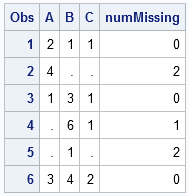It is the ARRAY statement that makes the CMISS function convenient. If the variables are contiguous in the data set (as in this example), you can use the double-dash notation (A -- C) to specify the variables. If your variables share a common prefix, you can use the colon wildcard character (:) to specify the variables. For other useful techniques to specify an array of variable names, see the SUGI 30 paper, "Techniques for Effectively Selecting Groups of Variables" by Stuart Pollack.

### Count missing values in each row in the SAS/IML language

In one of my first blog posts, I showed how to use the SAS/IML language to remove observations with missing values. However, that post was written prior to the release of SAS/IML 9.22, so now there is an easier way that uses the COUNTMISS function. The COUNTMISS function has an optional second parameter that determines whether the function returns the total number of missing values, the number in each column, or the number in each row. The following statements define a matrix with missing values and count the number of missing values in each row:

```proc iml; use Missing; read all var _NUM_ into x; close Missing;   rowMiss = countmiss(x, "ROW"); /* returns 0,1,2 or 3 for each row */ print rowmiss;```The output shows that the returned value is a vector with the same number of rows as x. The vector contains the number of missing values in each row of x.

It is now easy to use the LOC function (another function that I've written about often) to find the rows that contain missing values:

```/* which rows have one or more missing values? */ jdx = loc(rowMiss>0); print jdx;```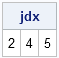Similarly, it is easy to extract the subset of rows that contain no missing values:

```idx = loc(rowMiss=0); x = x[idx,]; /* delete rows that contain a missing value */```

The x data matrix is the listwise deletion of the original data. You can now use the x matrix in statistical data analysis in PROC IML.After my post on detecting outliers in multivariate data in SAS by using the MCD method, Peter Flom commented "when there are a bunch of dimensions, every data point is an outlier" and remarked on the curse of dimensionality. What he meant is that most points in a high-dimensional cloud of points are far away from the center of the cloud.

### Distances and outliers in high dimensions

You can demonstrate this fact with a simulation. Suppose that you simulate 1,000 observations from a multivariate normal distribution (denoted MVN(0,Σ)) in d-dimensional space. Because the density of the distribution is highest near the mean (in this case, the origin), most points are "close" to the mean. But how close is "close"? You might extrapolate from your knowledge of the univariate normal distribution and try to define an "outlier" to be any point whose distance from the origin is more than some constant, such as five standardized units.

That sounds good, right? In one dimension, an observation from a normal distribution that is more than 5 standard deviations away from the mean is an extreme outlier. Let's see what happens for high-dimensional data. The following SAS/IML program does the following:

1. Simulates a random sample from the MVN(0,Σ) distribution.
2. Uses the Mahalanobis module to compute the Mahalanobis distance between each point and the origin. The Mahalanobis distance is a standardized distance that takes into account correlations between the variables.
3. Computes the distance of the closest point to the origin.

```proc iml; /* Helper function: return correlation matrix with "compound symmetry" structure: {v+v1 v1 v1, v1 v+v1 v1, v1 v1 v+v1 }; */ start CompSym(N, v, v1); return( j(N,N,v1) + diag( j(N,1,v) ) ); finish; load module=Mahalanobis; /* or insert definition of module here */   call randseed(12345); N = 1000; /* sample size */ rho = 0.6; /* rho = corr(x_i, x_j) for i^=j */ dim = T(do(5,200,5)); /* dim=5,10,15,...,200 */ MinDist = j(nrow(dim),1); /* minimum distance to center */ do i = 1 to nrow(dim); d = dim[i]; mu = j(d,1,0); Sigma = CompSym(d,1-rho,rho); /* get (d x d) correlation matrix */ X = randnormal(N, mu, Sigma); /* X ~ MVN(mu, Sigma) */ dist = Mahalanobis(X, mu, Sigma); MinDist[i] = min(dist); /* minimum distance to mu */ end;```

The following graph shows the distance of the closest point to the origin for various dimensions.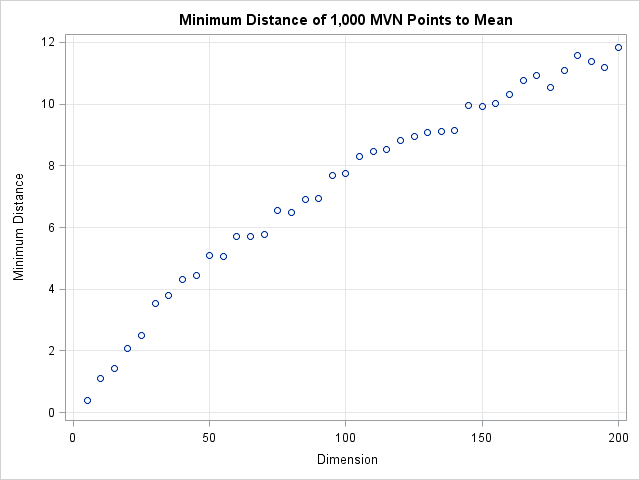The graph shows that the minimum distance to the origin is a function of the dimension. In 50 dimensions, every point of the multivariate normal distribution is more than 5 standardized units away from the origin. In 150 dimensions, every point is more than 10 standardized units away! Consequently, you cannot define outliers a priori to be observations that are more than 5 units away from the mean. If you do, you will, as Peter said, conclude that in 50 dimensions every point is an outlier.

### How to define an outlier cutoff in high dimensions

The resolution to this dilemma is to incorporate the number of dimensions into the definition of a cutoff value. For multivariate normal data in d dimensions, you can show that the squared Mahalanobis distances are distributed like a chi-square distribution with d degrees of freedom. (This is discussed in the article "Testing data for multivariate normality.") Therefore, you can use quantiles of the chi-square distribution to define outliers. A standard technique (which is used by the ROBUSTREG procedure to classify outliers) is to define an outlier to be an observation whose distance to the mean exceeds the 97.5th percentile. The following graph shows a the 97.5th percentile as a function of the dimension d. The graph shows that the cutoff distance is greater than the minimum distance and that the two distances increase in tandem.

`cutoff = sqrt(quantile("chisquare", 0.975, dim)); /* 97.5th pctl as function of dimension */`If you use the chi-square cutoff values, about 2.5% of the observations will be classified as outliers when the data is truly multivariate normal. (If the data are contaminated by some other distribution, the percentage could be higher.) You can add a few lines to the previous program in order to compute the percentage of outliers when this chi-square criterion is used:

```/* put outside DO loop */ PctOutliers = j(nrow(dim),1);/* pct outliers for chi-square d cutoff */ ... /* put inside DO loop */ cutoff = sqrt( quantile("chisquare", 0.975, d) ); /* dist^2 ~ chi-square */ PctOutliers[i] = sum(dist>cutoff)/N; /* dist > statistical cutoff */ ...```

The following graph shows the percentage of simulated observations that are classified as outliers when you use this scheme. Notice that the percentage of outliers is close to 2.5% independent of the dimension of the problem! By knowing the distribution of distances in high-dimensional MVN data, we are able to define a cutoff value that does not classify every point as an outlier.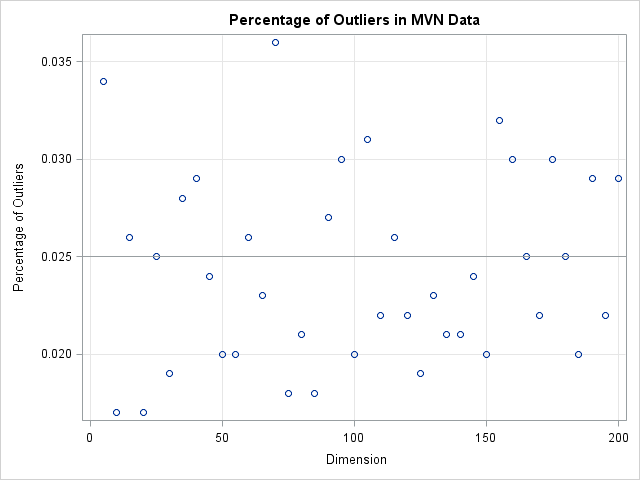To conclude, in high dimensions every data point is far away from the mean. If you use a constant cutoff value then you will erroneously classify every data point as an outlier. However, you can use statistical theory to define a reliable rule to detect outliers, regardless of the dimension of the data.A recent discussion on the SAS-L discussion forum concerned how to implement linear interpolation in SAS. Some people suggested using PROC EXPAND in SAS/ETS software, whereas others proposed a DATA step solution.

For me, the SAS/IML language provides a natural programming environment to implement an interpolation scheme. It also provides the flexibility to handle missing values and values that are outside of the range of the data. In this article I'll describe how to implement a simple interpolation scheme and how to modify the simple scheme to support missing values and other complications.

### What is interpolation?

There are many kinds of interpolation, but when most people use the term "interpolation" they are talking about linear interpolation. Given two points (x1,y1) and (x2,y2) and a value x such that x is in the interval [x1, x2], linear interpolation uses the point-slope formula from high-school algebra to compute a value of y such that (x,y) is on the line segment between (x1,y1) and (x2,y2). Linear interpolation between two points can be handled by defining the following SAS/IML module:

```proc iml; /* Interpolate such that (x,y) is on line segment between (x1,y1) and (x2,y2) */ start LinInterpPt(x1, x2, y1, y2, x); m = (y2-y1)/(x2-x1); /* slope */ return( m#(x-x1) + y1 ); /* point-slope formula */ finish;```

The following graph shows a linear interpolation scheme. The data are shown with round markers. The line segments are the graph of the piecewise-linear interpolation function for the data. Several points (shown with star-shaped markers) are interpolated. The next section shows how to compute the interpolated values.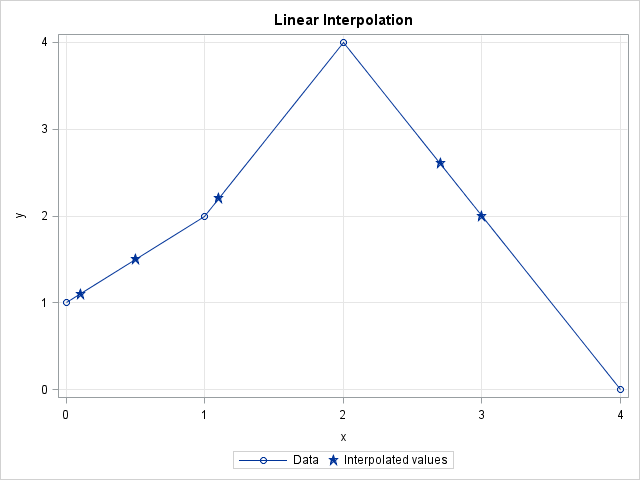### Linear interpolation: A simple function

Suppose you have a set of points (x1,y1), (x2,y2), ..., (xn,yn) that are ordered so that x1 < x2 < ... < xn. It is not difficult to use the LinearInterpPt function to interpolate a value, v in the interval [x1, xn): you simply find the value of k so that xk ≤ v < xk+1 and then call the LinInterpPt function to interpolate. If you have more than one value to interpolate, you can loop over the values, as shown in the following function:

```start Last(x); /* Helper module: return last element in a vector */ return( x[nrow(x)*ncol(x)] ); finish;   /* Linear interpolation: simple version */ start LinInterp1(x, y, v); /* Given column vectors (x, y), interpolate values for column vector v. Assume: 1. no missing values in x, y, or v 2. the values of x are unique and sorted 3. each element of v is in the interval [minX, maxX) */ fv = j(nrow(v),1); /* allocate return vector */ do i = 1 to nrow(v); k = Last( loc(x <= v[i] )); /* largest x less than v[i] */ fv[i] = LinInterpPt(x[k], x[k+1], y[k], y[k+1], v[i]); end; return( fv ); finish;   /* test it on some data */ xy = {0 1, 1 2, 2 4, 4 0 }; v = {0.1, 1.1, 0.5, 2.7, 3}; /* interpolate at these points */ fv = LinInterp1(xy[,1], xy[,2], v); print v fv;```The elements of v and fv are plotted as stars on the graph in the preceding section. The round markers are the data in the xy matrix.

### Linear interpolation: A more robust implementation

The LinearInterp1 function is short, simple, and does the job, but it makes a lot of assumptions about the structure of the (x,y) data and the values to be interpolated. In practice, it is more useful to have a function that makes fewer assumptions and also handles various error conditions. In particular, a useful function would do the following:

1. Handle the case where an element of v equals the maximum value xn.
2. Handle missing values in x or y by deleting those ordered pairs from the set of data values.
3. Check that the nonmissing values of x are unique and issue an error message if they are not. (Alternatively, you could combine repeated values by using the mean or median of the y values.)
4. Sort the ordered pairs by x.
5. Handle elements of v that are outside of interval [x1, xn]. The simplest scheme is to return missing values for these elements. I do not recommend extrapolation.
The first case is easily handled in the LinearInterp module. To implement the other cases, I'll write a module called Interp that handles cases 2–5 and then passes the remaining values of x, y, and v to the LinearInterp module. The following modules implement this strategy:
```start LinInterp(x, y, v); /* Assume x and v are sorted and x is unique. Return f(v; x,y) where f is linear interpolation function determined by (x,y) */ fv = j(nrow(v),1); /* allocate return vector */ begin = 1; /* set default value */ idx = loc(v=x); /* 1. handle v[i]=xMin separately */ if ncol(idx)>0 then do; fv[idx] = y; begin = Last(idx) + 1; end; do i = begin to nrow(v); /* remaining values have xMin < v[i] <= xMax */ k = Last( loc(x < v[i] )); /* largest x less than v[i] */ fv[i] = LinInterpPt(x[k], x[k+1], y[k], y[k+1], v[i]); end; return( fv ); finish;   start Interp(_x, _y, _v); /* Given column vectors (x, y), return interpolated values of column vector v */ /* 2. Delete missing values in x and y */ idx = loc( _x^=. & _y^=. ); if ncol(idx)<2 then return( j(nrow(_v),1,.) ); x = _x[idx]; y = _y[idx];   /* 3. check that the nonmissing values of x are unique */ u = unique(x); if nrow(x)^=ncol(u) then do; print "ERROR: x cannot contain duplicate values"; stop; /* Alternatively, use mean or median to handle repeated values */ end;   /* 4. sort by x */ xy = x || y; call sort(xy, 1); x = xy[,1]; y = xy[,2];   /* 5. don't interpolate outside of [minX, maxX]; delete missing values in v */ minX = x; maxX = Last(x); interpIdx = loc( _v>=minX & _v<=maxX & _v^=. ); if ncol(interpIdx)=0 then return( j(nrow(_v),1,.) );   /* set up return vector; return missing for v out of range */ fv = j(nrow(_v),1,.); fv[interpIdx] = LinInterp(x, y, _v[interpIdx] ); return (fv); finish;   /* test the modules */ xy = {0 1, . 3, 2 4, 4 0, 5 ., 1 2}; v = {0.1, 1.1, 0.5, 2.7, 3, ., 4, 5, -1}; /* interpolate at these points */ fv = Interp(xy[,1], xy[,2], v); print v fv;```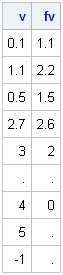The first five values of v are the same as in the previous section. The last four elements are values that would cause errors in the simple module.

Clearly it takes more work to handle missing values, unordered data, and out-of-range conditions. The simple function with six statements has evolved into two functions with about 30 statements. This is a classic example of "the programmer's 80-20 rule": In a typical program, only 20% of the statements are needed to compute the results; the other 80% handle errors and degenerate situations.

In spite of the extra work, the end result is worth it. I now have a robust module that performs linear interpolation for a wide variety of input data and interpolation values.Most statistical programmers have seen a graph of a normal distribution that approximates a binomial distribution. The figure is often accompanied by a statement that gives guidelines for when the approximation is valid. For example, if the binomial distribution describes an experiment with n trials and the probability of success for each trial is p, then the quantity np(1-p) must be larger than some cutoff (often 5 is used, but sometimes 10) in order for the approximation to be valid.

The following SAS/IML statements compute the binomial probabilities for n=50 and p=0.8 (so that np(1-p)=8) and for the approximating normal curve, which has mean np and variance np(1-p). These values are plotted by the SGPLOT procedure (SGPLOT statements not shown):

```proc iml; n = 50; /* number of trials: better approx for 100, 200, etc. */ p = 0.8; /* probability of success for each trial */ mu = n*p;/* classic approximation of binomial pdf by normal pdf */ stddev = sqrt(n*p*(1-p)); xMin = mu - 4*stddev; xMax = mu+4*stddev; x1 = T(floor(xMin):ceil(xMax)); /* evaluate binomial at integers */ y1 = pdf("Binom", x1, p, n); x2 = T(do(xMin, xMax, (xMax-xMin)/101)); y2 = pdf("Normal", x2, mu, stddev); /* write values to SAS data set; plot with SGPLOT */```The approximation looks good. However, the figure suggests that you need to be careful if you use a normal distribution to approximate extreme quantiles (such as 0.01 or 0.99) of the binomial distribution. Although the "middles" of the two distributions agree well, there appears to be les agreement in the tails. Also, recall that the quantiles of a discrete distribution are integers, which provides yet another source of error when approximating a binomial quantile with a normal quantile. This is evident when you overlay the normal CDF on the binomial CDF, as in the following figure:

```prob = T( do(0.01, 0.99, 0.005) ); q = quantile("binom", prob, p, n); /* ALWAYS an integer! */ qNormal = quantile("normal", prob, mu, stddev); diff = q - qNormal; /* error from approximating binomial quantiles */ /* write values to SAS data set; plot with SGPLOT */```The graph shows that there is considerable error for quantiles near zero and one. Is this important? It can be. For example, if you are creating a funnel plot for proportions the curves on the funnel plot are computed with the quantiles 0.001, 0.025, 0.975, and 0.999. These are extreme quantiles, but they are used to compute the funnel-like control limits, which are the most important feature of the plot. I suspect this is why David Spiegelhalter in his paper "Funnel plots for comparing institutional performance" used a rather complicated formula (in Appendix A.1.1) to interpolate the binomial quantiles in the funnel plot for proportions, rather than using a binomial approximation.

The following graph shows a close-up of the values of the binomial quantiles versus the normal approximation for the extreme quantiles near one. These are the values used to compute the upper control limits in a funnel plot. You can see that the normal approximation exhibits a systematic error, due to differences in the size of the binomial and normal tails.The lesson is this: even though it is common to use a normal distribution to approximate the binomial, the extreme quantiles of the distributions might not be close. Even when np(1-p) is fairly large, there are still sizeable differences in the values of the extreme quantiles of the distributions.SAS provides several ways to compute sample quantiles of data. The UNIVARIATE procedure can compute quantiles (also called percentiles), but you can also compute them in the SAS/IML language. Prior to SAS/IML 9.22 (released in 2010) statistical programmers could call a SAS/IML module that computes sample quantiles. With the release of SAS/IML 9.22, there is now a built-in QNTL call for computing sample quantiles.

### Computing quantiles

The QNTL call computes the samples quantiles for each row of a matrix. By default the 0.25, 0.5, and 0.75 quantiles are computed. (These are the 25th, 50th, and 75th percentiles, respectively.) The following statements compute sample quantiles for a matrix whose first column is from a standard normal distribution and whose second column is from a standard uniform distribution:

```proc iml; N = 1000; /* sample size */ u = j(N,1); z = j(N,1); /* allocate vectors */ call randseed(1); call randgen(u, "Uniform"); call randgen(z, "Normal"); x = z || u; /* concatenate into 1000 x 2 matrix */   call qntl(q, x); /* by default, compute Q1, median, and Q3 */ print q;```The output shows that the sample quantiles of the columns are close to the theoretical quantiles for the underlying populations, as shown by the following statements:

```/* compute quantiles of distributions */ qNormal = quantile("Normal", {0.25, 0.5, 0.75}); qUnif = quantile("Uniform", {0.25, 0.5, 0.75}); print qNormal qUnif;```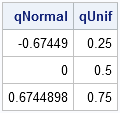Notice that sample quantiles of data are computed by using the QNTL call, but theoretical quantiles of a "named" distribution are computed by using the QUANTILE function in Base SAS.

### Computing other quantiles

The QNTL call supports a third parameter with which you can specify specific quantiles. For example, the following statements compute the 5th, 10th, 90th, and 95th quantiles of each column of x:

```p = {0.05, 0.10, 0.90, 0.95}; call qntl(q, x, p); /* compute 5th, 10th, 90th, and 95th quantiles */```

### Labeling quantiles

The output from the QNTL call is a matrix, q. The number of columns of q is the number of n columns of x, and each row represents a quantile. If you want to display the quantiles, it is convenient to use the CHAR function or the PUTN function to form row labels that indicate the quantiles or percentiles, as shown in the following statements:

```labels = "P" + putn(100*p, "Z2."); /* concat "P" and {"05" "10" "90" "95"} */ varNames = {"Normal" "Uniform"}; print q[rowname=labels colname=VarNames];```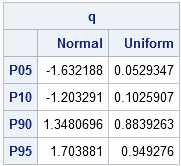As shown in the example, the Zw.d format can be useful for converting the numerical quantiles to percentile labels.I work with continuous distributions more often than with discrete distributions. Consequently, I am used to thinking of the quantile function as being an inverse cumulative distribution function (CDF). (These functions are described in my article, "Four essential functions for statistical programmers.")

For discrete distributions, they are not. To quote from my "Four essential functions" article: "For discrete distributions, the quantile is the smallest value for which the CDF is greater than or equal to the given probability." (Emphasis added.)

There is a simple numerical way to examine the relationship between the quantile and CDF: call one function after the other and see if the resulting answer is the same value that you started with. (In other words, compose the functions to see if they are the identity function.) The following SAS/IML statements compute a normal quantile, followed by a CDF:

```proc iml; /* the quantile function is the inverse CDF for continuous distributions */ prob = 0.8; /* start with 0.8 */ q = quantile("Normal", prob); /* compute normal quantile z_0.8 */ cdf = cdf("Normal", q); /* compute CDF(z_0.8) */ print prob q cdf; /* get back to 0.8 */```As expected, the QUANTILE function and the CDF function are inverse operations for a continuous distribution such as the normal distribution. However, this is not true for discrete distributions such as the binomial distribution:

```/* the quantile function is NOT the inverse CDF for discrete distributions */ prob = 0.8; q = quantile("Binomial", prob, 0.5, 10); /* q = 80th pctl of Binom(p,n) */ cdf = cdf("Binomial", q, 0.5, 10); /* CDF(q) does NOT equal 0.8 */ print prob q cdf;```The reason becomes apparent by looking at the CDF function for the binomial distribution. Consider 10 tosses of a fair coin that has probability p=0.5 of landing on "heads." The Binom(0.5, 10) distribution models this experiment. The CDF function displays the probability that the ten tosses will result in m heads, for m=0, 1, ..., 10, as shown in the following graph:

```data BinomCDF(drop=p N); p = 0.5; N = 10; do m = 0 to N by 0.1; cdf = cdf("Binomial", m, p, N); output; end; run;   proc sgplot data=BinomCDF; title "Probability of m heads in 10 coin tosses"; title2 "CDF of Binom(0.5, 10)"; scatter x=m y=cdf; xaxis label="Number of Heads" values=(0 to 10) grid; yaxis label="P(Number of heads <= m)" grid; run;```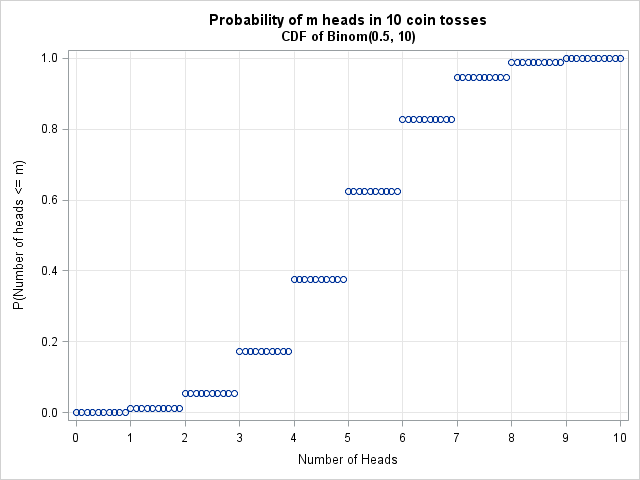The CDF function is a step function that maps an entire interval to a single probability. For example, the entire interval [5, 6) is mapped to the value 0.623. The quantile function looks similar and maps intervals to the integers 0, 1, ..., 9, 10. For example, the binomial quantile of x is 5 for every x in the interval (0.377, 0.623). This example generalizes: the quantile for a discrete distribution always returns a discrete value.

A consequence of this fact was featured in my article on "Funnel plots for proportions." Step 3 of creating a funnel plot is complicated because it computes a continuous approximation to discrete control limits that arise from binomial quantiles. If you approximate the binomial distribution by a normal distribution, Step 3 becomes simpler to implement, but the funnel curves based on normal quantiles are different from the curves based on binomial quantiles. A future article will explore how well the normal quantiles approximate binomial quantiles.As a SAS developer, I am always looking ahead to the next release of SAS. However, many SAS customer sites migrate to new releases slowly and are just now adopting versions of SAS that were released in 2010 or 2011. Consequently, I want to write a few articles that discuss recent additions to the SAS/IML language, where "recent" goes back a few years. For the several Mondays, my "Getting Started" articles will review SAS/IML language features that were added in SAS/IML 9.22 (released in 2010) and SAS/IML 9.3 (released in 2011).

Today's topic: basic descriptive statistics for sample data. In particular, the MEAN, VAR, and STD functions.

### The MEAN function: Much more than sample means

Prior to SAS/IML 9.22, statistical programmers used the colon (:) subscript reduction operator to compute the arithmetic mean of data. For example, the following SAS/IML program computes the grand mean, the row means, and the column means of data in a 5x2 matrix:

```proc iml; x = {-1 -1, 0 1, 1 2, 1 0, -1 0 };   rowMeans = x[ ,:]; colMeans = x[:, ]; grandMean= x[:]; print x rowMeans, colMeans grandMean;```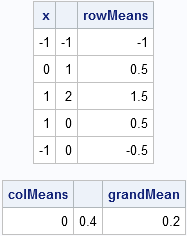The MEAN function was introduced in SAS/IML 9.22. The expression mean(x) computes the arithmetic mean of each column of a matrix. It is equivalent to x[:,]. The MEAN function also supports trimmed and Winsorized means, which are robust estimators of location.

Because the MEAN function computes the arithmetic mean of each column of a matrix, you need to be careful when computing the mean of a vector. Make sure that the function argument it is a column vector, not a row vector. For example, the following statement does NOT compute the mean of the elements in the vector, g:

```g = 1:5; /* row vector {1 2 3 4 5} */ m = mean(g); /* probably not what you want! */```

Instead, use the transpose function (T) or the COLVEC function so that the argument to the MEAN function is a column vector:

`m = mean(colvec(g)); /* correct */`

A previous article discusses the trimmed and Winsorized means and provides an example.

### The VAR function for computing the sample variance

Prior to SAS/IML 9.22, statistical programmers could use a module to compute the sample variance of each column of a matrix. The VAR function is more efficient, but the results are the same. The following statement computes the sample variance of each column of x:

```v = var(x); print v;```If you compute the variance of data in a vector, make sure that you pass a column vector to the VAR function.

### The STD function for computing the sample standard deviation

The STD function (introduced in SAS 9.3) is simply the square root of the sample variance. As such, the STD function is merely a convenient shortcut for sqrt(var(x)):

```s = std(x); print s;```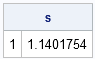Once again, if you compute the standard deviation of data in a vector, make sure that you pass a column vector to the STD function.I've blogged several times about multivariate normality, including how to generate random values from a multivariate normal distribution. But given a set of multivariate data, how can you determine if it is likely to have come from a multivariate normal distribution?

The answer, of course, is to run a goodness-of-fit (GOF) test to compare properties of the data with theoretical properties of the multivariate normal (MVN) distribution. For univariate data, I've written about the usefulness of the quantile-quantile (Q-Q) plot to model the distribution of data, and it turns out that there is a similar plot that you can use to assess multivariate normality. There are also analytic GOF tests that can be used.

To see how these methods work in SAS, we need data. Use the RANDNORMAL function in SAS/IML software to generate data that DOES come from a MVN distribution, and use any data that appears nonnormal to examine the alternative case. For this article, I'll simulate data that is uniformly distributed in each variable to serve as data that is obviously not normal. The following SAS/IML program simulates the data:

```proc iml; N = 100; /* 100 obs for each distribution */ call randseed(1234);   /* multivariate normal data */ mu = {1 2 3}; Sigma = {9 1 2, 1 6 0, 2 0 4 }; X = randnormal(N, mu, Sigma);   /* multivariate uniform data */ v = j(N, ncol(mu)); /* allocate Nx3 matrix*/ call randgen(v, "Uniform"); /* each var is U[0,1] */ v = sqrt(12)*(v - 1/2); /* scale to mean 0 and unit variance */ U = mu + T(sqrt(vecdiag(Sigma))) # v; /* same mean and var as X */```

### A graphical test of multivariate normality

If you want a quick check to determine whether data "looks like" it came from a MVN distribution, create a plot of the squared Mahalanobis distances versus quantiles of the chi-square distribution with p degrees of freedom, where p is the number of variables in the data. (For our data, p=3.) As I mentioned in the article on detecting outliers in multivariate data, the squared Mahalanobis distance has an approximate chi-squared distribution when the data are MVN. See the article "What is Mahalanobis distance?" for an explanation of Mahalanobis distance and its geometric interpretation.

I will use a SAS/IML function that computes Mahalanobis distances. You can insert the function definition into the program, or you can load the module from a SAS catalog if it was previously stored. The following program computes the Mahalanobis distance between the rows of X and the sample mean:

```load module=Mahalanobis; /* or insert module definition here */   Mean = mean(X); /* compute sample mean and covariance */ Cov = cov(X); md = mahalanobis(X, Mean, Cov);```

For MVN data, the square of the Mahalanobis distance is asymptotically distributed as a chi-square with three degrees of freedom. (Note: for a large number of variables you need a very large sample size before the asymptotic chi-square behavior becomes evident.) To plot these quantities against each other, I use the same formula that PROC UNIVARIATE uses to construct its Q-Q plots, as follows:

```md2 = md##2; call sort(md2, 1); s = (T(1:N) - 0.375) / (N + 0.25); chisqQuant = quantile("ChiSquare", s, ncol(X));```

If you plot md2 versus chiSqQuant, you get the graph on the left side of the following image. Because the points in the plot tend to fall along a straight line, the plot suggests that the data are distributed as MVN. In contrast, the plot on the right shows the same computations and plot for the uniformly distributed data. These points do not fall on a line, indicating that the data are probably not MVN. Because the samples contain a small number of points (100 for this example), you should not expect a "perfect fit" even if the data are truly distributed as MVN.### Goodness-of-fit tests for multivariate normality

Mardia's (1974) test multivariate normality is a popular GOF test for multivariate normality. Mardia (1970) proposed two tests that are based definitions of multivariate skewness and kurtosis. (See von Eye and Bogat (2004) for an overview of this and other methods.) It is easy to implement these tests in the SAS/IML language.

However, rather than do that, I want to point out that SAS provides the %MULTNORM macro that implements Mardia's tests. The macro also plots the squared Mahalanobis distances of the observations to the mean vector against quantiles of a chi-square distribution. (However, it uses the older GPLOT procedure instead of the newer SGPLOT procedure.) The macro requires either SAS/ETS software or SAS/IML software. The following statements define the macro and call it on the simulated MVN data:

```/* write data from SAS/IML to SAS data set */ varNames = "x1":"x3"; create Normal from X[c=varNames]; append from X; close Normal; quit;   /* Tests for MV normality */ %inc "C:\path of macro\multnorm.sas"; %multnorm(data=Normal, var=x1 x2 x3, plot=MULT);```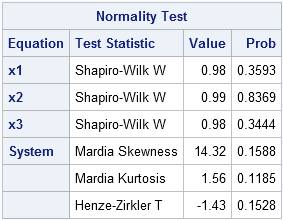The macro generates several tables and graphs that are not shown here. The test results shown in the preceding table indicate that there is no reason to reject the hypothesis that the sample comes from a multivariate normal distribution. In addition to Mardia's test of skewness and kurtosis, the macro also performs univariate tests of normality on each variable and another test called the Henze-Zirkler test.

### Another graphical tool: Plot of marginal distributions

To convince yourself that the simulated data are multivariate normal, it is a good idea to use the SGSCATTER procedure to create a plot of the univariate distribution for each variable and the bivariate distribution for each pair of variables. Alternatively, you can use the CORR procedure as is shown in the following statements. The CORR procedure can also produce the sample mean and sample covariance, but these tables are not shown here.

```/* create scatter plot matrix of simulated data */ proc corr data=Normal COV plots(maxpoints=NONE)=matrix(histogram); var x:; ods select MatrixPlot; run;```The scatter plot matrix shows (on the diagonal) that each variable is approximately normally distributed. The off-diagonal elements show that the pairwise distributions are bivariate normal. This is characteristic of multivariate normal data: all marginal distributions are also normal. (This explains why the %MULTNORM macro includes univariate tests of normality in its test results.) Consequently, the scatter plot matrix is a useful graphical tool for investigating multivariate normality.